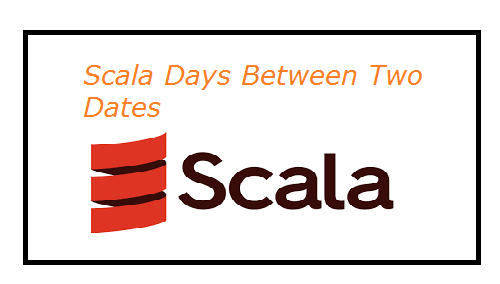Trending Articles       10 Flutter Listview Examples       Flutter Custom Button Example       Flutter Audio Recording       Flutter 2.0 Autocomplete TextField       Current Location Google Maps

# Scala - Number of Days between Two dates

Last updated Jun 03, 2021

In this scala programming example we will calculate number of days between two dates. For this we will use scala date class object. In this first we will take two date class objects with defined two dates. Then we will get the time in milliseconds by date class method getTime(), then subtract two dates time. Finally we will get the number of days by converting time in millisecondsto days.

In the example we will import date class

 `import java.util.Date;`Example code for calculate number of days between two dates in scala

 import java.util.Date; object Sample {   def main(args: Array[String]) {     var firstDate = new Date(2021, 6, 1);     var secondDate = new Date(2022, 1, 1);     var ms = secondDate.getTime() - firstDate.getTime();     var days = (ms / (60 * 60 * 24 * 1000));     println("Days: " + days);   } }

This will print the number of days between two dates 2021-6-1 and 2021-1-1 is

`Days: 215`

 Article Contributed By :100 Views